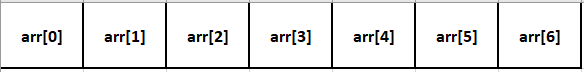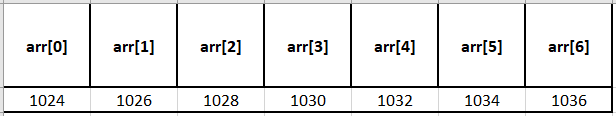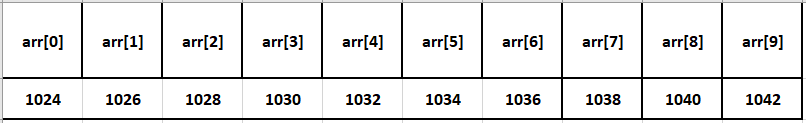In this tutorial, we are going to learn about Arrays in C.

In a programming language, the data that has to be processed is loaded in memory and held in variables. When we want to process an integer value, we declare an int variable and assign a value to it.

Assume we want to write a program that prints the total marks scored by students in a class (say, for a total of 35 students).

One way of doing it is to declare 35 int variables, which is not scalable when we think of multiple classes or a complete school.

In such cases, when we want to store multiple values of the same data typeC provides us a derived data type called an array.

An array is an ordered sequence of finite data items of the same data type and that shares a common name. The common name is the array name and each individual data item is known as an element of the array.

The elements of the array are stored in contiguous memory locations starting from a memory location allocated/stored in the array variable.

For example, an array of size 10 (int arr;) can be visualized as shown below.Essentially an array can be thought of as a sequence of buckets. The first bucket is identified with the number 0, the second bucket with 1, and so on. This number is called the index (or) subscript.

For example, if we want to store a value 10 at the first index, the code is arr = 10;.
Similarly, if we want to store a value 20 in the second bucket, the code will be arr = 20; and so on.
If we want to store a value 70 in the last bucket, the code will be arr = 70;.

An element of an array is retrieved/accessed using its index. For example, the value stored in the first bucket can be printed using the below code:

``printf("%d", arr); // remember that array's index starts from 0 and not 1``

There are one-dimensional and multi-dimensional arrays.

one-dimensional array can be used to represent a list of data elements and is also known as a vector.

two-dimensional array is used to represent a table of data items consisting of rows and columns and is also known as a matrix.

A three-dimensional array can be used to represent a collection of pages.

The syntax for declaring a one-dimensional array is given below:

``````data_type array_name[size]; // a single array is declared
data_type array_name_1[size_1], array_name_2[size_2]....array_name_n[size_n];
//multiple arrays are declared in the same line
``````

Here, data_type refers to the data type of the elements in the array and it can be a primitive data type.
array_name_1, array_name_2, …..etc refers to the identifiers which represent the array names.
size is an integer expression representing the total number of elements in the array.

Let us consider an example,

``int arr;``

The above one-dimensional array declaration defines an integer array by name num of size 7, meaning it represents a block of 7 consecutive storage locations that store int values.

Here each element in the array can be accessed by num, num, num, num, num, num, num, where 0, 1, 2, 3, 4, 5, 6 represent the subscripts or indices of the respective elements in the array.Each element in an array can be accessed by the name of the array followed by the subscript or directly by the address. The array variable holds the base address of that entire array.

Let us consider an example declaration of a int array of size 10.

``int num;``

Each element in the array can be accessed by num, num,…., num, where 0, 1, 2,..,9 represents subscripts or indices of the elements in the array.In the above example, the array variable num contains the base address of the entire array. Let us assume the base address as some random number: 1024.

Whenever 1 is added to the array variable num, it gives the next location of the array i.e.,

``````num + 1 is same as &num
1024 + 1 = 1024 + 1 * [scale factor] = 1024 + 1 * 2 = 1024 + 2 = 1026
``````

In the above line, 2 is the size of data type int in 16-bit machines. 2 is also referred to as the scale factor.

The formula for finding the ith location of the array element is

`` element = base_address + i * scale_factor``

The scale factor is automatically calculated by the system, which is a value representing the size of the data type of the array.

Note: In the below program, the symbol * in *(a + i) is used to find the value at the given address. We will learn more about it while learning about pointers in the later sections.

## REFERENCES:

Happy Learning### About the Author: Hemanth Krishna Vineel RamayanamI’m an Undergraduate Student at the Indian Institute of Information Technology, Design, and Manufacturing, Kurnool, Andhra Pradesh. I belong to the CSE domain.